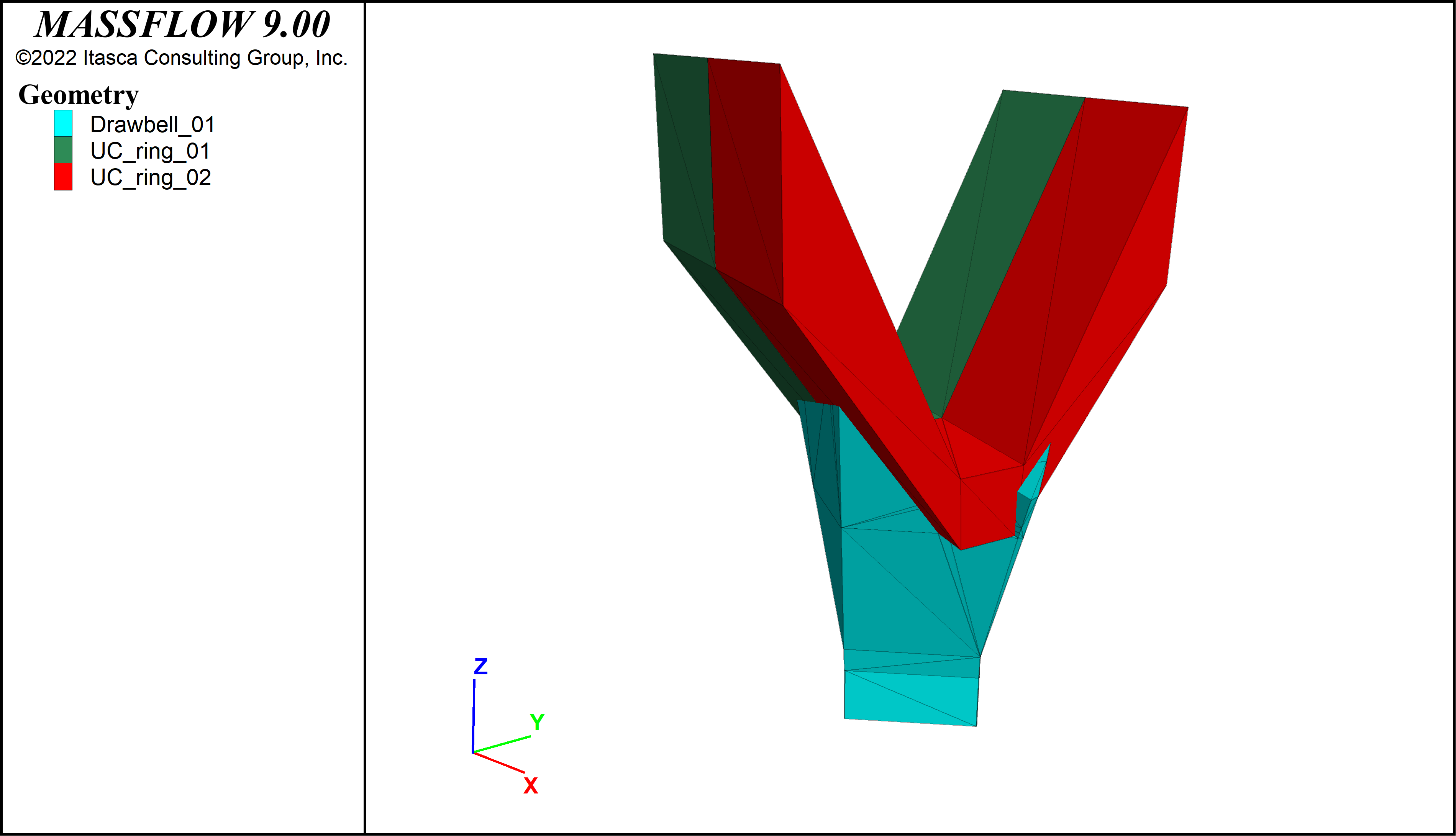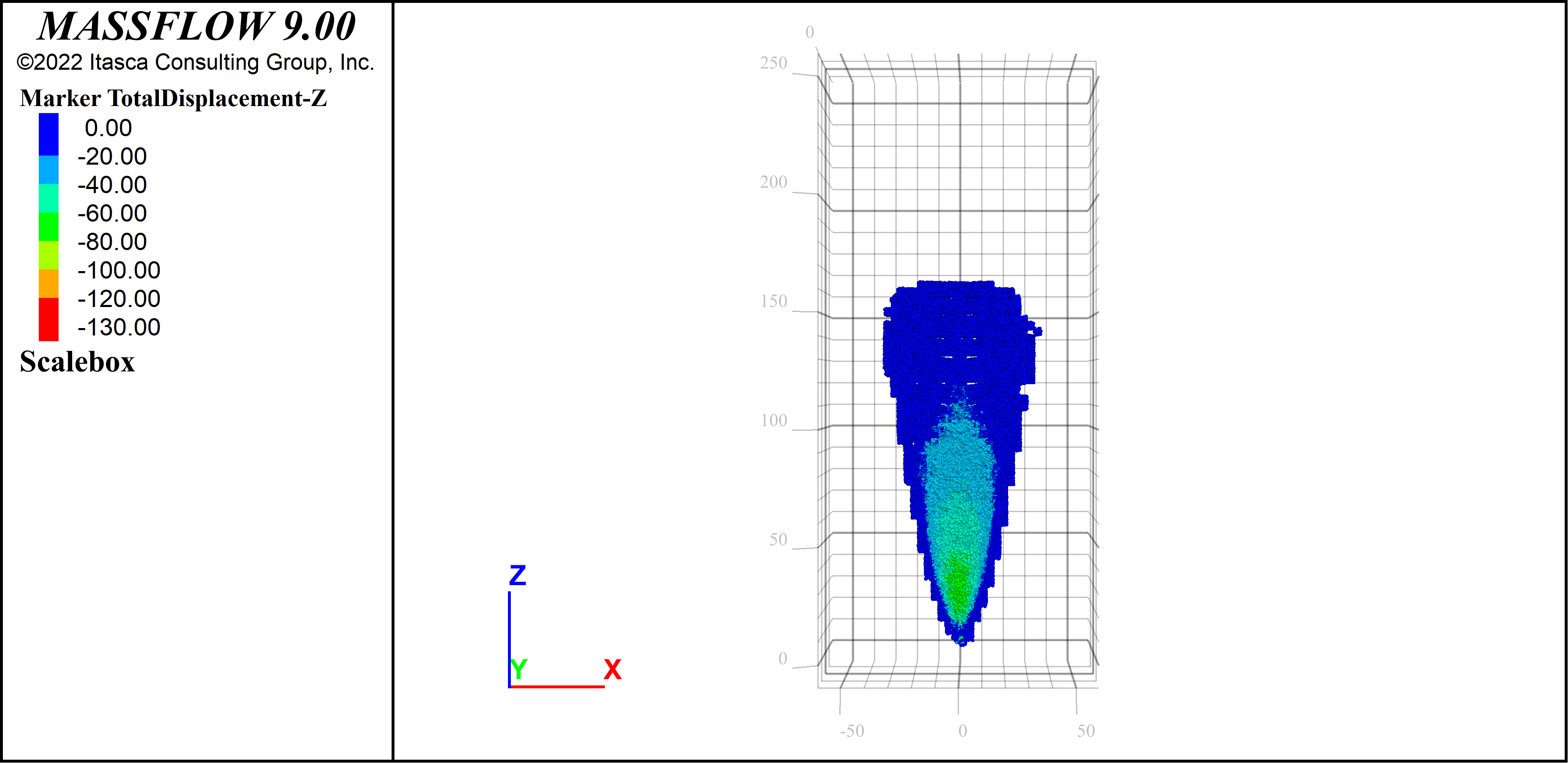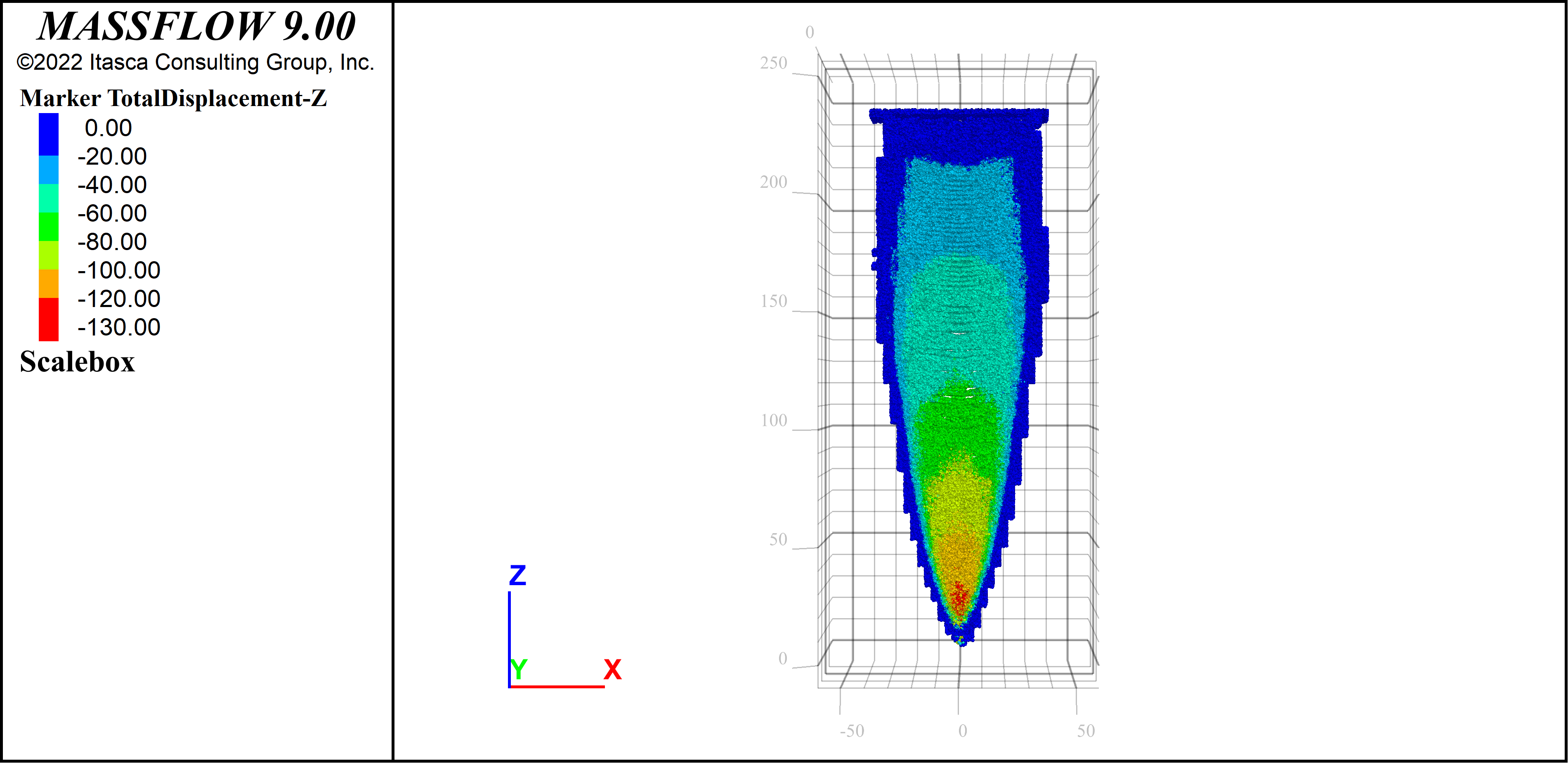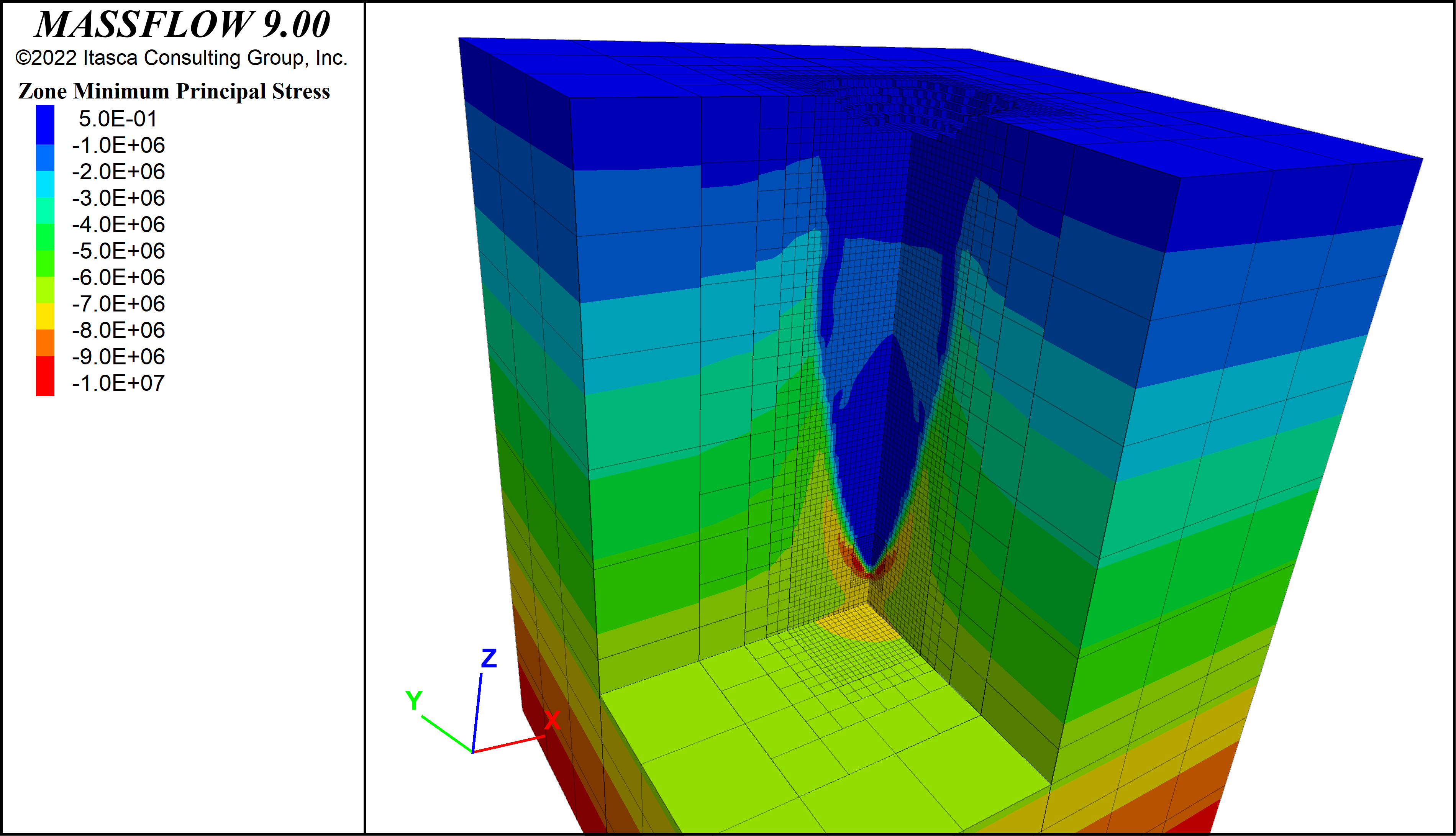# Massflow Coupling Example

Problem Statement

Note

To view this projects in MassFlow, use the menu command Help ► Examples…. Choose “MassFlow/ Examples/ Coupling/ ” and select coupled.prj. The data files used is shown at the end of this example. All data and input files are found in the project directory.

This example demonstrates how to couple a FLAC3D mechanical analysis with a MassFlow flow analysis. The example is fairly simple, using only one drawpoint, but it illustrates the basic coupling techniques. You will need both a FLAC3D and MassFlow license to run this example. The entire example can be run either with MassFlow or FLAC3D: both programs contain the necessary components of the other to facilitate a coupled analysis.

Building the Model

## Initial Geometry

The FLAC3D zones are set up in the input data file “01_geometry.dat”. First, a new model state is specified, a title for the model is given and three geometry surfaces are imported to show the drawbell and UC Rings (???).

model new
model title 'Couple Massflow-FLAC3D'

geom import 'data/Drawbell_01.stl'
geom import 'data/UC_ring_01.stl'
geom import 'data/UC_ring_02.stl'


The geometries can be plotted and are shown in Figure 1.Figure 1: Geometry surfaces imported for the coupled analysis.

Next a small FISH function is defined to specify the size of the MassFlow model and calculate the corresponding size of the FLAC3D model. It is assumed that the FLAC3D model should be larger than the MassFlow model and should extend past the edges of the MassFlow model by a factor of 3 times the massflow model size, except in the vertical direction, where the massflow model is a factor of 5 bigger. FLAC3D zones are then generated according to these calculated dimensions.

fish define input_

; half dimension of massflow model
block1=48.0

; FLAC3D model extends past massflow model
x1 = -3*block1
x2 = 3*block1
y1 = x1
y2 = x2
z1 = -3*block1  ; negative
z2 = 5*block1 ; positive
end
[input_]

zone create brick size 6 6 8 ...
point 0 [x1] [y1] [z1] ...
point 1 [x2] [y1] [z1] ...
point 2 [x1] [y2] [z1] ...
point 3 [x1] [y1] [z2]


The zones that overlap the MassFlow model are then identified and densified

; identify massflow part
zone group 'massflow' range pos-x [-block1] [block1] ...
pos-y [-block1] [block1] ...
pos-z [-0.5*block1] [5*block1]

; densify massflow part
zone densify gradient-limit maximum-length [block1/16] repeat range group 'massflow'


Finally, the zones are densified further around the drawpoint and then all zones are attached and the model is saved. The final zone geometry is as shown in Figure 2.

; densify around drawpoint
zone densify range pos-x -12 12 pos-y -12 12 pos-z -6 24

zone attach by-face

model save 'saves/01_Geometry'Figure 2: FLAC3D zones. Part of the model is cut away for visualization purposes.

## Properties and Initial Conditions

The rock proerties, boundary conditions and initial conditions are set up in the file “02_initial.dat”.

The rock is assigned a Hoek-Brown constutitive model with a GSI of 30. Hoek-Brown strength parameters are calculated according to Hoek (1994) and stiffness parameters according to Hoek and Diederichs (2006).

Side boundaries are fixed perpendicular to the boundary faces, the bottom boundary is fixed in all directions, and the top boundary is free.

Gravity is turned on, initial stresses due to gravity are installed and the model is solved to equilibrium.

## Mining

The cave mining procedure is outlined in “03_CaveMining.dat”. Firstly, it is assumed that material inside of the rings and the drawbell have failed and so are assigned a mohr-coulomb consitutive model with residual properties. Stresses in these zones are also set to 0. The model is then solved to equilibrium.

The MassFlow part of the model is then set up. Mine blocks, drawpoints and the draw schedule are imported. The slice thickness of the Isolated Movement Zone (IMZ) is set to 0.5 of the mine block size, and the marker spacing is set to 0.25 m. These values will give medium resolution results. For a faster model runs use a larger size and spacing, for more detailed results, use smaller spacings. A marker report is set to be dumped out every period (7 days). The save frequency is set to a large number since the model will be saved by hand every period. The commands used to set up the massflow model are shown below. The massflow model geometry is shown in Figure 3.

massflow mine-block import 'data/BM_Simple_3m_Weibull.txt'
massflow drawpoint import "data/DP_Massflow_simple.txt"
massflow drawpoint import-drawbell "data/DB_Massflow_simple.txt"
massflow drawpoint import-drawperiod "data/DS_Massflow_simple.txt"
massflow initialize layer-thickness 0.5 ;medium
massflow marker initialize spacing 0.25 ;medium

; we will save by hand, so set save period to large number
massflow initialize percent-empty 0.75 save-period [tot_periods+1]
massflow marker report-period 1
massflow marker filename 'dumps/massflow'
massflow cleanFigure 3: Mine blocks and drawbell in the MassFlow model. A quarter of the mine block model is cut away for visualization purposes.

A FISH function is then defined that sets up a loop over the number of desired periods (156 in this case). Before the loop, the zones inside of each mine block are stored by calling the function “massflow_ini”. This function and others described below can be found in “FishSupport.fis”. The function then enters the loop. Each period, the following steps occur:

1. Each mine block is checked to see if it contains any zones that are failing, or have been set to “Mobilized” (see below). If the mineblock contains failing or mobilized zones, it is set to be flowable (the period of flow is set to the current period).
2. Any null zones are set to be “Mobilized Active” and are assigned mohr-coulomb constitutive model with residual properties.
3. The MassFlow computation is done for one period (7 days).
4. Markers are read and FLAC3D zones are set to be “Mobilized Active”, “Mobilized Inactive” or “Air” depending on the markers that they contain.
5. Flowable zones are assigned residual properties and 0 stress. Non-flowable zones are frozen and the model is solved.

Results

The propagation of the caved material is examined by looking at results after 50 weeks (~ 1 year) and the full three years of simulation. Figure 4 and Figure 5 show the state of the mine blocks after 1 year and 3 years. It is clear how the mobilized zone is propagating up the model until it reaches the surface after 3 years.

The day at which the different mineblocks were mobilized can be seen by contouring by the dynamic period (Figure 6). Recall, that the dynamic periods were set using FISH when the underlying zones failed. You can see how the period at which the mineblocks were able to flow progresses upwards.

The markers after 3 years are shown in Figure 7 and cross sections showing marker vertical displacements are shown in Figure 8 and Figure 9. The displacements patterns are interesting, showing significant movement near the edges of the IMZ, but less movement elsewhere.

Finally, the maximum compressive stress contours for the FLAC3D zones are shown in Figure 10 and Figure 11. The stress in the mobilized zone is very low as expected (since we set the stresses to 0 at the start of each period), however it is interesting to observe the stress concentrations below the drawpoint and the stress redistributions around the mobilized zone.Figure 8: A cross-section of markers showing vertical displacements after 50 weeks.Figure 9: A cross-section of markers showing vertical displacements after 3 years.Figure 10: Maximum compressive stresses in zones after 50 weeks.Figure 11: Maximum compressive stresses in zones after 3 years.

References

Hoek E. Strength of rock and rock masses. ISRM News Journal 1994;2(2):4-16.

Hoek E, Diederichs MS. Empirical estimation of rock mass modulus. International Journal of Rock Mechanics and Mining Sciences 2006;43(2):203-15.

Data Files

01_geometry.dat

model new
model title 'Couple Massflow-FLAC3D'

geom import 'data/Drawbell_01.stl'
geom import 'data/UC_ring_01.stl'
geom import 'data/UC_ring_02.stl'

fish define input_

; half dimension of massflow model
block1=48.0

; FLAC3D model extends past massflow model
x1 = -3*block1
x2 = 3*block1
y1 = x1
y2 = x2
z1 = -3*block1  ; negative
z2 = 5*block1 ; positive
end
[input_]

zone create brick size 6 6 8 ...
point 0 [x1] [y1] [z1] ...
point 1 [x2] [y1] [z1] ...
point 2 [x1] [y2] [z1] ...
point 3 [x1] [y1] [z2]

; identify massflow part
zone group 'massflow' range pos-x [-block1] [block1] ...
pos-y [-block1] [block1] ...
pos-z [-0.5*block1] [5*block1]

; densify massflow part
zone densify gradient-limit maximum-length [block1/16] repeat range group 'massflow'

; densify around drawpoint
zone densify range pos-x -12 12 pos-y -12 12 pos-z -6 24

zone attach by-face

model save 'saves/01_Geometry'


02_initial.dat

model rest 'saves/01_Geometry'

; Hoek-Brown parameters
[global ucs_=35e6]
[global gsi_=30.]
[global mi_=15.]
[global ei_=40.e9]
[global dens_=2700.]
[global a_hb = 0.5+1./6.*(math.exp(-gsi_/15)-math.exp(-20./3.))]
[global s_hb = math.exp((gsi_-100)/9)]
[global m_hb = mi_*math.exp((gsi_-100)/28)
[global E_ = ei_*(0.02+1./(1+math.exp((60-gsi_)/11)))]
[global poiss_ = 0.32-0.0015*gsi_]

zone cmodel assign hoek-brown
zone prop dens [dens_] young [E_] poiss [poiss_] ...
constant-a [a_hb] constant-mb [m_hb] constant-s [s_hb] ...
constant-sci [ucs_] constant-dilation 10 flag-brittle true

; boundary conditions
zone face apply vel-x 0 range pos-x [x1]
zone face apply vel-x 0 range pos-x [x2]
zone face apply vel-y 0 range pos-y [y1]
zone face apply vel-y 0 range pos-y [y2]
zone face apply vel 0 0 0 range pos-z [z1]

; initial conditions
model gravity 9.81
zone initialize-stresses

model history mechanical unbalanced-maximum
model large-strain off
model solve elastic convergence 1
model save 'saves/02_Initial'


03_CaveMining.dat

model restore 'saves/02_Initial'

zone gridpoint initialize disp 0 0 0
zone initialize state 0

fish define massflow_inputs
;DEFINE DEFAULT BROKEN ROCK MASS
global targetvsi_=0.67 ;equal to the max vsi used in the model
global coh_res=0
global fri_res=42
global dil_res=0
global ten_res=0
global you_res=300e6
global poi_res=0.2
global den_res=dens_/(1.+targetvsi_)

global tot_periods=156 ;3 years
end
[massflow_inputs]

;BLASTED Drawbell and UCL RINGS BEFORE CAVE MINING
;blasted zones
zone group 'UCL_01' slot 'Initial' range geometry-space 'UC_ring_01' inside
zone group 'UCL_02' slot 'Initial' range geometry-space 'UC_ring_02' inside
zone group 'Drawbell' slot 'Initial' range geometry-space 'Drawbell_01' inside

zone cmodel mohr-coulomb range group 'UCL_01' or 'UCL_02' or 'Drawbell'
zone property density [den_res] young [you_res] poisson [poi_res] ...
cohesion [coh_res] friction [fri_res] tension [ten_res] dilation [dil_res] ...
range group 'UCL_01' or 'UCL_02' or 'Drawbell'

zone initialize stress xx 0 yy 0 zz 0  xy 0 xz 0 yz 0 ...
range group 'UCL_01' or 'UCL_02' or 'Drawbell'
model solve convergence 1
model save 'saves/03_Blasted_Initial'

==============================================================
;SET UP MASSFLOW

model restore 'saves/03_Blasted_Initial'

call 'FishSupport.fis'

massflow mine-block import 'data/BM_Simple_3m_Weibull.txt'
massflow drawpoint import "data/DP_Massflow_simple.txt"
massflow drawpoint import-drawbell "data/DB_Massflow_simple.txt"
massflow drawpoint import-drawperiod "data/DS_Massflow_simple.txt"
massflow initialize layer-thickness 0.5 ;medium
massflow marker initialize spacing 0.25 ;medium

; we will save by hand, so set save period to large number
massflow initialize percent-empty 0.75 save-period [tot_periods+1]
massflow marker report-period 1
massflow marker filename 'dumps/massflow'
massflow clean

fish define run_FLAC3D_MASSFLOW

; initialize data structures
massflow_ini

loop local n_(1,tot_periods)

if n_ # 1
; set some mineblocks to flowable
update_CPED(n_)

; change air zones to active mobilized
NullToMobilized
endif
command
massflow compute period 1
endcommand

; read markers and set zone flowable states

; change zone properties and solve
Apply_CC

; save every 10 periods
if n_ % 10 = 0
local sav_= 'saves/run_'+string(n_)
command
model save [sav_]
endcommand
endif
endloop
end
[run_FLAC3D_MASSFLOW]

; save final state
model save 'saves/run_3years'


FishSupport.fis

fish auto-create off

fish define massflow_ini
;
; Initialize some data structures for coupled analysis
;
massflow.mineblock.extra(::massflow.mineblock.list) = list
loop foreach local zp zone.list
if zone.group(zp) = 'massflow'
local mb = massflow.mineblock.containing(zone.pos(zp))
if mb # null
massflow.mineblock.extra(mb)('end') = zp
endif
endif
end_loop

; define marker states
global UNBULKED_IMZ = -2
global UNBULKED_COLLAPSE = -1
global UNBULKED_RILLING = 0
global PARTIALLY_BULKED = 1
global FULLY_BULKED = 2
global AIR = 3

; define extra variable offsets for zones
global NUM_ACTIVE = 1
global NUM_INACTIVE = 2
global NUM_AIR = 3
end

====================================================
;
; Read markers and set zone flow states
;
command
zone group 'None' slot 'Flow State'
zone initialize extra [NUM_ACTIVE] 0
zone initialize extra [NUM_INACTIVE] 0
zone initialize extra [NUM_AIR] 0
endcommand

; count number of each moving marker type in each zone
loop foreach local mk massflow.marker.list
global v_ = massflow.marker.pos(mk)
global type_ = massflow.marker.type(mk)

; skip extracted
if massflow.marker.extracted(mk)
continue
endif

if type_ > 1 ;<= 1 are markers created to fill the blocks only
local zp=zone.containing(v_) ;zone containing the marker
if zp = null
continue
endif

if type_ = AIR
zone.extra(zp,NUM_AIR) += 1
else if massflow.marker.active(mk)
zone.extra(zp,NUM_ACTIVE) += 1
else
zone.extra(zp,NUM_INACTIVE) += 1
endif
endif
endloop

; figure out zone type from number of marker types
loop foreach local zi zone.list
if zone.model(zi) # 'null' ;Non_Fill group
if zone.group(zi)='massflow'
local count_=math.max(zone.extra(zi,NUM_ACTIVE),zone.extra(zi,NUM_INACTIVE),zone.extra(zi,NUM_AIR))
if count_ # 0 ;it is a zone containing a marker
if count_ = zone.extra(zi,NUM_AIR) then
zone.group(zi,'Flow State')='Air'
endif
if count_=zone.extra(zi,NUM_INACTIVE) then
zone.group(zi,'Flow State')='Mobilized Inactive'
endif
if count_=zone.extra(zi,NUM_ACTIVE) then
zone.group(zi,'Flow State')='Mobilized Active'
endif
endif
endif
endif
endloop
end
======================================================
fish define Apply_CC
;
; Set flowable zones to residual properties
; Freeze non-flowable zones and solve
;
command
zone gridpoint initialize displacement 0 0 0 range group 'Mobilized Active'

;active flowable zones
zone cmodel assign mohr-coulomb range group 'Mobilized Active' cmodel 'null' not
zone initialize density [den_res] range group 'Mobilized Active' cmodel 'null' not
zone property young [you_res] poisson [poi_res] cohesion [coh_res] friction [fri_res] ...
tension [ten_res] dilation [dil_res] range group 'Mobilized Active' cmodel 'null' not

zone cmodel assign mohr-coulomb range group 'Air' cmodel 'null' not
zone initialize density [den_res] range group 'Air' cmodel 'null' not
zone property young [you_res] poisson [poi_res] cohesion [coh_res] friction [fri_res] ...
tension [ten_res] dilation [dil_res] range group 'Air' cmodel 'null' not

zone gridpoint initialize velocity 0 0 0

zone gridpoint fix vel range group 'Mobilized Active' not

;stresses are zeroed in the mobilized rock
zone initialize stress xx 0 yy 0 zz 0  xy 0 xz 0 yz 0 range group 'Mobilized Active'

; Don't use convergence 1.
; There may be failing blocks that don't equilibrate
model solve

zone gridpoint free vel range group 'Mobilized Active' not
zone gridpoint initialize velocity  0 0 0

; reapply boundary conditions
zone face apply vel-x 0 range pos-x [x1]
zone face apply vel-x 0 range pos-x [x2]
zone face apply vel-y 0 range pos-y [y1]
zone face apply vel-y 0 range pos-y [y2]
zone face apply vel 0 0 0 range pos-z [z1]

zone relax excavate step 500 range group 'Air'

; Don't use convergence 1.
; There may be failing blocks that don't equilibrate
model solve ratio 1e-5 or cycles 5000
end_command
end
====================================================
fish define NullToMobilized
COMMAND
; we need to temporarily assign a group because
; once we change to mohr-coulomb, we can't use RANGE CMODEL NULL
zone group 'None' slot 'temp'
zone group 'NulltoMobilized' slot 'temp' range cmodel 'null'
zone cmodel mohr-coulomb range group 'NulltoMobilized'
zone initialize density [den_res] range group 'NulltoMobilized'

zone property young [you_res] poisson [poi_res] cohesion [coh_res] friction [fri_res] &
tension [ten_res] dilation [dil_res] range group 'NulltoMobilized'

zone group  'Mobilized Active' slot 'Flow State' range group 'NulltoMobilized'
END_COMMAND
end
============================================
fish define update_CPED(current_period)
; check if there are any flowable zones inside the mineblock
loop foreach local mb massflow.mineblock.list
local flowable = false
loop foreach local zi massflow.mineblock.extra(mb)
if zone.group(zi,'Flow State') # 'None'
flowable = true
endif
if zone.state(zi) > 0
flowable = true
endif
end_loop

if flowable
if massflow.mineblock.period.dyn(mb) > current_period
massflow.mineblock.period.dyn(mb) = current_period
endif
endif
endloop
end

fish auto-create on